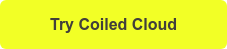# How to Convert a pandas Dataframe into a Dask Dataframe

July 27, 2021

In this post, we will cover:

• How (and when) to convert a pandas DataFrame into a Dask DataFrame;
• Demonstrating 2x (or more!) speedup with an example;
• Discuss some best practices.

pandas is a very powerful Python library for manipulating and analyzing structured data, but it has some limitations. pandas does not leverage parallel computing capabilities of modern systems and throws a MemoryError when you try to read larger-than-memory data. Dask can help solve these limitations. Dask is a library for parallel and distributed computing in Python. It provides a collection called Dask DataFrame that helps parallelize the pandas API.

You can follow along with the code in this notebook!

## Analyzing library data with pandas

Let’s start with a sample pandas workflow of reading data into a pandas DataFrame and doing a groupby operation. We read a subset of the Seattle Public Library checkouts dataset (~4GB) and find the total number of items checked out based on the ‘Usage Class’ (i.e., physical vs. digital).

```df = pd.read_csv("checkouts-subset.csv") #

df.groupby("UsageClass").Checkouts.sum() # ~1.2 seconds
```

The computation takes about 1.2 seconds on my computer. Can we speed this up using Dask DataFrame?

To leverage single-machine parallelism for this analysis, we can convert the pandas DataFrame into a Dask DataFrame. A Dask DataFrame consists of multiple pandas DataFrames split across an index as shown in the following diagram:

We can use Dask’s from_pandas function for this conversion. This function splits the in-memory pandas DataFrame into multiple sections and creates a Dask DataFrame. We can then operate on the Dask DataFrame in parallel using its pandas-like interface. Note that we need to specify the number of partitions or the size of each chunk while converting.

`ddf = dd.from_pandas(df, npartitions=10)`

This conversion might take a minute, but it’s a one-time cost. We can now perform the same computation as earlier:

```ddf.groupby("UsageClass").Checkouts.sum().compute() # ~679 ms
seconds```

This took ~680 milliseconds on my machine. That’s more than twice as fast as pandas!

It’s important to note here that Dask DataFrame provides parallelism, but it may not always be faster than pandas. For example, operations that require sorting take more time because data needs to be moved between different sections. We suggest sticking with pandas if your dataset fits in memory.

If you work with sizable data, it is likely stored on a remote system across multiple files, and not on your local machine. Dask allows you to read this data in parallel, which can be much faster than reading with pandas.

```import dask.dataframe as dd

Both pandas and Dask also support several file-formats, including Parquet and HDF5, data formats optimized for scalable computing. You can check out the list of formats in the pandas documentation.

Dask DataFrame uses the single-machine threaded scheduler by default, which works well for local workflows. Dask also provides a distributed scheduler with a lot more features and optimizations. It can be useful even for local development. For instance, you can take advantage of Dask’s diagnostic dashboards. To use the distributed scheduler, use:

```from dask.distributed import Client
client = Client()```

```import dask.dataframe as dd